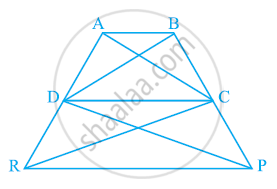# In the given figure, ar (DRC) = ar (DPC) and ar (BDP) = ar (ARC). Show that both the quadrilaterals ABCD and DCPR are trapeziums. - Mathematics

In the given figure, ar (DRC) = ar (DPC) and ar (BDP) = ar (ARC). Show that both the quadrilaterals ABCD and DCPR are trapeziums.#### Solution

It is given that

Area (ΔDRC) = Area (ΔDPC)

As ΔDRC and ΔDPC lie on the same base DC and have equal areas, therefore, they must lie between the same parallel lines.

∴ DC || RP

Therefore, DCPR is a trapezium.

It is also given that

Area (ΔBDP) = Area (ΔARC)

⇒ Area (BDP) − Area (ΔDPC) = Area (ΔARC) − Area (ΔDRC)

⇒ Area (ΔBDC) = Area (ΔADC)

Since ΔBDC and ΔADC are on the same base CD and have equal areas, they must lie between the same parallel lines.

∴ AB || CD

Therefore, ABCD is a trapezium.

Concept: Corollary: Triangles on the same base and between the same parallels are equal in area.
Is there an error in this question or solution?

#### APPEARS IN

NCERT Class 9 Maths
Chapter 9 Areas of Parallelograms and Triangles
Exercise 9.3 | Q 16 | Page 164